# Colleges with the lowest SAT scores in Tennessee

Top 10 colleges in Tennessee with the lowest SAT scores
Looking for the colleges with the lowest SAT scores in Tennessee? Well you're in luck! We've compiled a national college database and have created a list of the top 10 universities with the lowest SAT scores in Tennessee below. If you are not a good test taker or worried about your test scores, this list is for you. These are the schools whose applicants had the lowest average SAT scores in Tennessee, which means that you can get into these colleges with a lower SAT score. We also include each college's ACT scores and acceptance rate so that you can see where you would have the easiest time getting in. Read on to find out more.

## The LeMoyne-Owen College SAT scores

The average SAT score for The LeMoyne-Owen College is 890.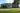The average SAT score of 890 breaks down into:

• SAT math: 445

The average ACT score for The LeMoyne-Owen College is 16 and their acceptance rate is 100%.

## Tennessee State University SAT scores

The average SAT score for Tennessee State University is 980.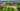The average SAT score of 980 breaks down into:

• SAT math: 490

The average ACT score for Tennessee State University is 18 and their acceptance rate is 51.8%.

## Tennessee Wesleyan College SAT scores

The average SAT score for Tennessee Wesleyan College is 1030.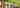The average SAT score of 1030 breaks down into:

• SAT math: 510

The average ACT score for Tennessee Wesleyan College is 22 and their acceptance rate is 57.7%.

## Cumberland University SAT scores

The average SAT score for Cumberland University is 1050.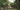The average SAT score of 1050 breaks down into:

• SAT math: 520

The average ACT score for Cumberland University is 22 and their acceptance rate is 46.4%.

## King University SAT scores

The average SAT score for King University is 1050.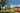The average SAT score of 1050 breaks down into:

• SAT math: 510

The average ACT score for King University is 20 and their acceptance rate is 58%.

## East Tennessee State University SAT scores

The average SAT score for East Tennessee State University is 1060.The average SAT score of 1060 breaks down into:

• SAT math: 540

The average ACT score for East Tennessee State University is 23 and their acceptance rate is 87.1%.

## Austin Peay State University SAT scores

The average SAT score for Austin Peay State University is 1071.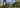The average SAT score of 1071 breaks down into:

• SAT math: 520

The average ACT score for Austin Peay State University is 22 and their acceptance rate is 93.8%.

## Carson-Newman University SAT scores

The average SAT score for Carson-Newman University is 1078.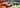The average SAT score of 1078 breaks down into:

• SAT math: 530

The average ACT score for Carson-Newman University is 22 and their acceptance rate is 66%.

## Fisk University SAT scores

The average SAT score for Fisk University is 1090.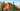The average SAT score of 1090 breaks down into:

• SAT math: 530

The average ACT score for Fisk University is 21 and their acceptance rate is 80.9%.

## The University of Memphis SAT scores

The average SAT score for The University of Memphis is 1098.The average SAT score of 1098 breaks down into:

• SAT math: 530